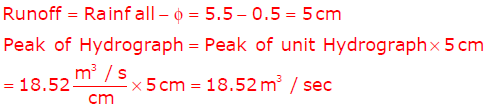# Civil Engineering - CE 2012 GATE Paper (Practice Test)

## 65 Questions MCQ Test Mock Test Series for Civil Engineering (CE) GATE | Civil Engineering - CE 2012 GATE Paper (Practice Test)

Description
This mock test of Civil Engineering - CE 2012 GATE Paper (Practice Test) for GATE helps you for every GATE entrance exam. This contains 65 Multiple Choice Questions for GATE Civil Engineering - CE 2012 GATE Paper (Practice Test) (mcq) to study with solutions a complete question bank. The solved questions answers in this Civil Engineering - CE 2012 GATE Paper (Practice Test) quiz give you a good mix of easy questions and tough questions. GATE students definitely take this Civil Engineering - CE 2012 GATE Paper (Practice Test) exercise for a better result in the exam. You can find other Civil Engineering - CE 2012 GATE Paper (Practice Test) extra questions, long questions & short questions for GATE on EduRev as well by searching above.
QUESTION: 1

### Q. 1 – Q. 5 carry one mark each. Q.1 Choose the most appropriate alternative from the options given below to complete the following sentence: Despite several ––––––––– the mission succeeded in its attempt to resolve the conflict.

Solution:

Setback means a reversal or hindrance in progress. The sentence wants to express that despite several hindrances the mission was successful in its objective.

QUESTION: 2

### The cost function for a product in a firm is given by 5q2, where q is the amount of production. The firm can sell the product at a market price of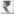50 per unit. The number of units to be produced by the firm such that the profit is maximized is

Solution: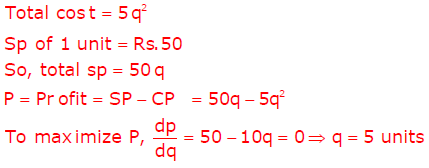QUESTION: 3

### Choose the most appropriate alternative from the options given below to complete the following sentence: Suresh’s dog is the one ––––––––– was hurt in the stampede.

Solution:

Who and whom are used for people and human beings.
That is used when the part following it cannot be avoided and the sentence will be incomplete without it.
E.g. Suresh Dog is the one that was hurt in the stampede.
Which is used for the sentence which is not necessary to include in the sentence,
E.g. Diamonds, which are expensive, often elicit forgiveness.

QUESTION: 4

Choose the grammatically INCORRECT sentence:

Solution: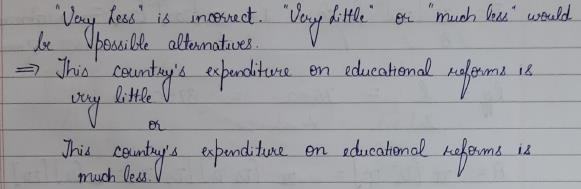QUESTION: 5

Which one of the following options is the closest in meaning to the word given below?
Mitigate

Solution:

Mitigate means making (something bad) less severe, serious or painful. Therefore, diminish is a synonym of mitigate.

QUESTION: 6

Q. 6 - Q. 10 carry two marks each.

Q.6 A political party orders an arch for the entrance to the ground in which the annual convention is being held. The profile of the arch follows the equation y = 2x – 0.1x2 where y is the height of the arch in meters. The maximum possible height of the arch is

Solution: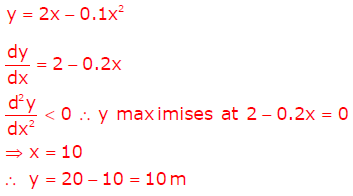QUESTION: 7

Wanted Temporary, Part-time persons for the post of Field Interviewer to conduct personal interviews to collect and collate economic data. Requirements: High School-pass, must be available for Day, Evening and Saturday work. Transportation paid, expenses reimbursed.

Which one of the following is the best inference from the above advertisement?

Solution:

There is no mention of gender in the question. Thus, one can conclude only at the time of interview whether the job is gender discriminatory or not.
Xenophobic means having or showing a dislike of or prejudice against people from other countries. However, no such statement is also made.
Thus, eliminating the options it can be concluded that the advertisement is not designed to make the post attractive.

QUESTION: 8

Given the sequence of terms, AD CG FK JP, the next term is

Solution: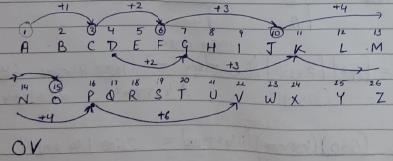QUESTION: 9

Which of the following assertions are CORRECT?
P: Adding 7 to each entry in a list adds 7 to the mean of the list
Q: Adding 7 to each entry in a list adds 7 to the standard deviation of the list
R: Doubling each entry in a list doubles the mean of the list
S: Doubling each entry in a list leaves the standard deviation of the list unchanged

Solution: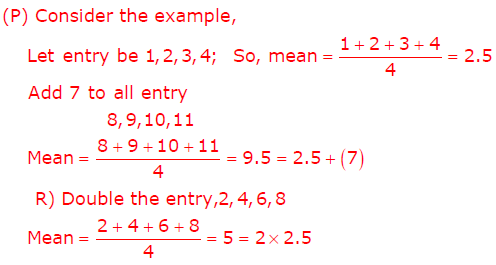QUESTION: 10

An automobile plant contracted to buy shock absorbers from two suppliers X and Y. X supplies 60% and Y supplies 40% of the shock absorbers. All shock absorbers are subjected to a quality test. The ones that pass the quality test are considered reliable. Of X’s shock absorbers, 96% are reliable. Of Y’s shock absorbers, 72% are reliable.

The probability that a randomly chosen shock absorber, which is found to be reliable, is made by Y is

Solution: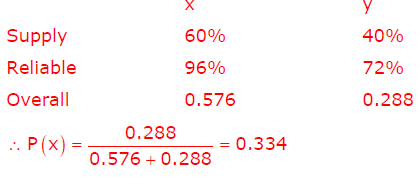QUESTION: 11

Q. 11 – Q. 35 carry one mark each.

The estimate of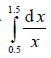obtained using Simpson’s rule with three-point function evaluation exceeds the exact value by

Solution: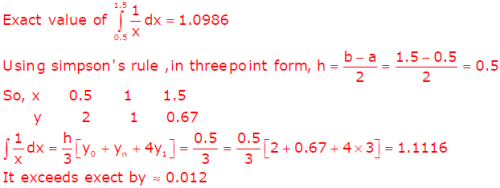QUESTION: 12

The annual precipitation data of a city is normally distributed with mean and standard deviation as 1000 mm and 200 mm, respectively. The probability that the annual precipitation will be more than 1200 mm is

Solution: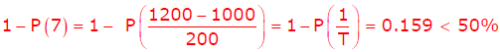QUESTION: 13

The infinite series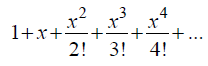corresponds to

Solution: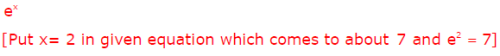QUESTION: 14

The Poisson’s ratio is defined as

Solution: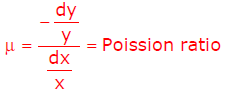QUESTION: 15

The following statements are related to bending of beams:

I The slope of the bending moment diagram is equal to the shear force.
II The slope of the shear force diagram is equal to the load intensity.
III The slope of the curvature is equal to the flexural rotation.
IV The second derivative of the deflection is equal to the curvature.

The only FALSE statement is

Solution: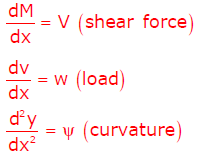QUESTION: 16

If a small concrete cube is submerged deep in still water in such a way that the pressure exerted on all faces of the cube is p, then the maximum shear stress developed inside the cube is

Solution: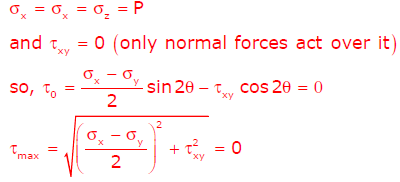QUESTION: 17

As per IS 456:2000, in the Limit State Design of a flexural member, the strain in reinforcing bars under tension at ultimate state should not be less than

Solution: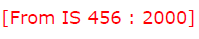QUESTION: 18

Which one of the following is categorised as a long-term loss of prestress in a prestressed concrete member?

Solution:

 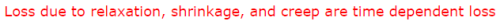QUESTION: 19

In a steel plate with bolted connections, the rupture of the net section is a mode of failure under

Solution:

Steel plate with bolted section may fail in following three cases-
A. Gross yielding of section
B. Net Tension Rupture if the Section
C. Block Shear Failure

QUESTION: 20

The ratio of the theoretical critical buckling load for a column with fixed ends to that of another column with the same dimensions and material, but with pinned ends, is equal to

Solution: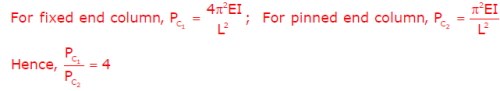QUESTION: 21

The effective stress friction angle of a saturated, cohesionless soil is 38°. The ratio of shear stress to normal effective stress on the failure plane is

Solution: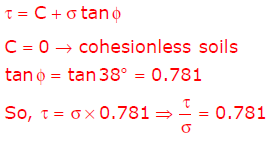QUESTION: 22

Two series of compaction tests were performed in the laboratory on an inorganic clayey soil
employing two different levels of compaction energy per unit volume of soil. With regard to the
above tests, the following two statements are made.

I The optimum moisture content is expected to be more for the tests with higher energy.
II The maximum dry density is expected to be more for the tests with higher energy.

The CORRECT option evaluating the above statements is

Solution: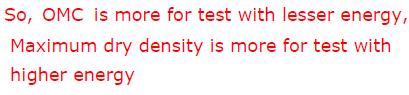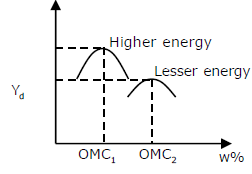QUESTION: 23

As per the Indian Standard soil classification system, a sample of silty clay with liquid limit of 40% and plasticity index of 28% is classified as

Solution: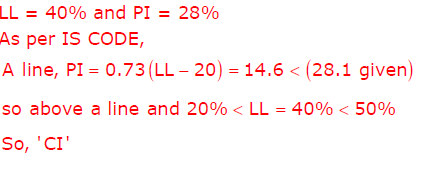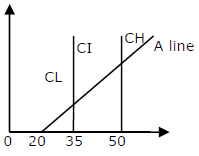QUESTION: 24

A smooth rigid retaining wall moves as shown in the sketch causing the backfill material to fail. The backfill material is homogeneous and isotropic, and obeys the Mohr-Coulomb failure criterion. The major principal stress is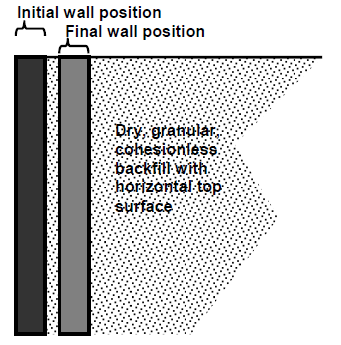Solution:

The question provides all the assumptions of Rankine Theory’s of earth pressure in the statement like smooth wall surface, dry cohesionless sand, vertical retaining wall.
Moreover, it is the case of horizontal backfill without any surcharge. As the wall move towards the soil thus, it will lead to the development of passive earth pressure acting normal to the wall face.

QUESTION: 25

An embankment is to be constructed with a granular soil (bulk unit weight = 20 kN/m3) on a saturated clayey silt deposit (undrained shear strength = 25 kPa). Assuming undrained general shear failure and bearing capacity factor of 5.7, the maximum height (in m) of the embankment at the point of failure is

Solution:
QUESTION: 26

A trapezoidal channel is 10.0 m wide at the base and has a side slope of 4 horizontal to 3 vertical. The bed slope is 0.002. The channel is lined with smooth concrete (Manning’s n = 0.012). The hydraulic radius (in m) for a depth of flow of 3.0 m is

Solution: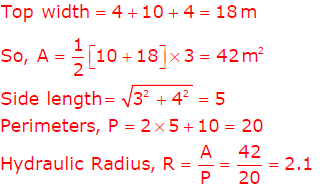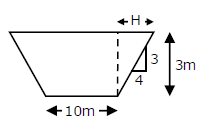QUESTION: 27

A rectangular open channel of width 5.0 m is carrying a discharge of 100 m3/s. The Froude number of the flow is 0.8. The depth of flow (in m) in the channel is

Solution: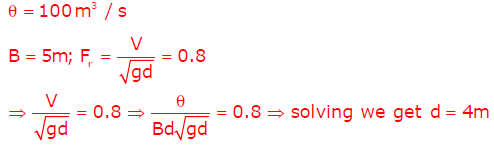QUESTION: 28

The circular water pipes shown in the sketch are flowing full. The velocity of flow (in m/s) in the branch pipe “R” is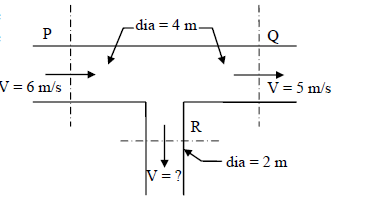Solution: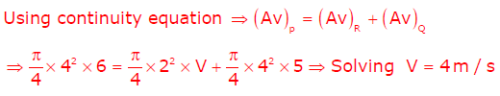QUESTION: 29

The ratio of actual evapo-transpiration to potential evapo-transpiration is in the range

Solution:

Potential Evapotranspiration is the maximum possible evapotranspiration had there been no shortage of water.
Actual Evapotranspiration is the actual evapotranspiration taking place in present moisture conditions.
Actual Evapotranspiration <= Potential Evapotranspiration
Thus, ratio varies from 0 to 1.

QUESTION: 30

A sample of domestic sewage is digested with silver sulphate, sulphuric acid, potassium dichromate and mercuric sulphate in chemical oxygen demand (COD) test. The digested sample is then titrated with standard ferrous ammonium sulphate (FAS) to determine the un-reacted amount of

Solution:
QUESTION: 31

Assertion [a]: At a manhole, the crown of the outgoing sewer should not be higher than the crown of the incoming sewer.
Reason [r]: Transition from a larger diameter incoming sewer to a smaller diameter outgoing sewer at a manhole should not be made.

The CORRECT option evaluating the above statements is :

Solution: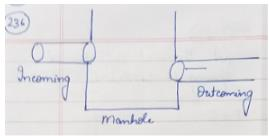The outgoing sewer should always be lower than the incoming sewer for free flow (nor the sewer will flow back to the incoming sewer pipe).
The diameter of the outgoing sewer should be equal to or greater than the diameter of incoming sewer to avoid any clogging situation..
Thus, both the statements are correct but the reason does not explain the statement.

QUESTION: 32

Two major roads with two lanes each are crossing in an urban area to form an un-controlled intersection. The number of conflict points when both roads are one-way is “X” and when both roads are two-way is “Y”. The ratio of X to Y is

Solution: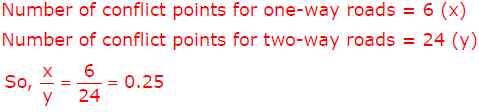QUESTION: 33

Two bitumen samples “X” and “Y” have softening points 45°C and 60°C, respectively. Consider the following statements:

I. I Viscosity of “X” will be higher than that of “Y” at the same temperature.
II Penetration value of “X” will be lesser than that of “Y” under standard conditions.

The CORRECT option evaluating the above statements is

Solution: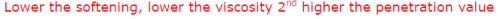QUESTION: 34

Solution:

The instrument can be used for direct roughness measurement or for calibrating other equipment like Bump Integrator. In RHD Merlin is used for calibrating purpose. It consists of a metal frame 1.8 meters long with a wheel at the front, a foot at the rear and a probe midway between them which rests on the road surface.

QUESTION: 35

Which of the following errors can be eliminated by reciprocal measurements in differential leveling?

I Error due to earth’s curvature
II Error due to atmospheric refraction

Solution:

Reciprocal Levelling involves keeping the instrument at A and taking reading of B and vice versa. Thus, the method eliminates any possibility of error due to the inclined line of collimation, earth’s curvature and atmospheric refraction.

QUESTION: 36

Q. 36 - Q. 65 carry two marks each.

The error in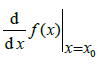for a continuous function estimated with h = 0.03 using the central difference formula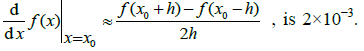The values of x0 and f(x0) are 19.78 and 500.01, respectively. The corresponding error in the central difference estimate for h = 0.02 is approximately

Solution:
QUESTION: 37

In an experiment, positive and negative values are equally likely to occur. The probability of obtaining at most one negative value in five trials is

Solution: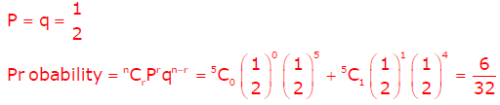QUESTION: 38

The eigenvalues of matrix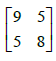are

Solution: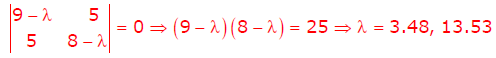QUESTION: 39

For the parallelogram OPQR shown in the sketch,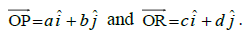The area of the parallelogram is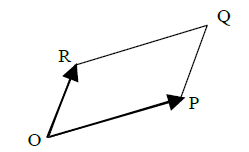Solution:QUESTION: 40

The solution of the ordinary differential equation dy/dx+2y=0 for the boundary condition, y = 5 at x = 1 is

Solution: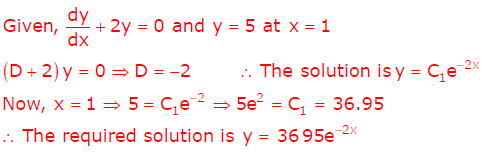QUESTION: 41

A simply supported beam is subjected to a uniformly distributed load of intensity w per unit length, on half of the span from one end. The length of the span and the flexural stiffness are denoted as l and EI, respectively. The deflection at mid-span of the beam is

Solution: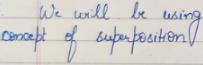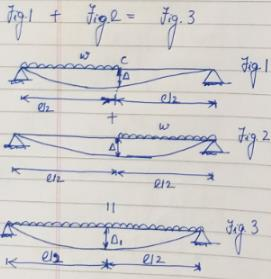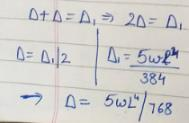QUESTION: 42

The sketch shows a column with a pin at the base and rollers at the top. It is subjected to an axial force P and a moment M at mid-height. The reaction(s) at R is/are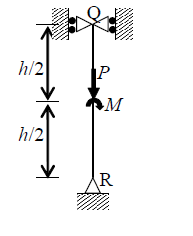Solution: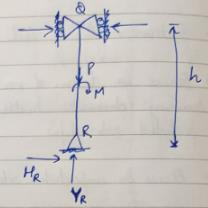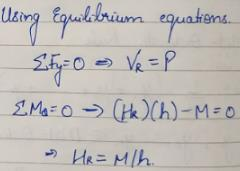QUESTION: 43

A concrete beam prestressed with a parabolic tendon is shown in the sketch. The eccentricity of the tendon is measured from the centroid of the cross-section. The applied prestressing force at service is 1620 kN. The uniformly distributed load of 45 kN/m includes the self-weight.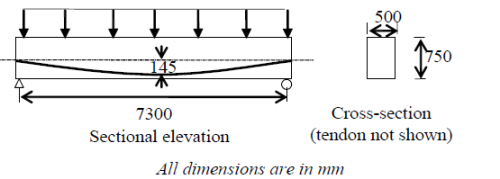The stress (in N/mm2) in the bottom fibre at mid-span is

Solution: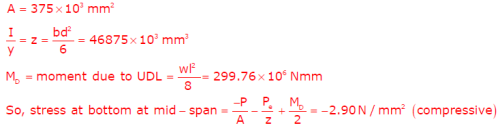QUESTION: 44

A symmetric frame PQR consists of two inclined members PQ and QR, connected at ‘Q’ with a rigid joint, and hinged at ‘P’ and ‘R’. The horizontal length PR is l. If a weight W is suspended at ‘Q’, the bending moment at ‘Q’ is

Solution: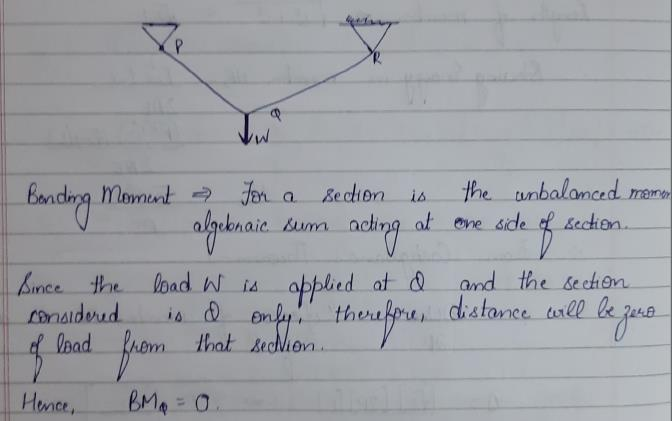QUESTION: 45

Two plates are connected by fillet welds of size 10 mm and subjected to tension, as shown in the sketch. The thickness of each plate is 12 mm. The yield stress and the ultimate tensile stress of steel are 250 MPa and 410 MPa, respectively. The welding is done in the workshop (λmw = 1.25). As per the Limit State Method of IS 800:2007, the minimum length (rounded off to the nearest higher multiple of 5 mm) of each weld to transmit a force P equal to 270 kN is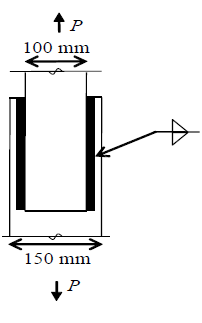Solution:
QUESTION: 46

Two soil specimens with identical geometric dimensions were subjected to falling head permeability tests in the laboratory under identical conditions. The fall of water head was measured after an identical time interval. The ratio of initial to final water heads for the test involving the first specimen was 1.25. If the coefficient of permeability of the second specimen is 5-times that of the first, the ratio of initial to final water heads in the test involving the second specimen is

Solution: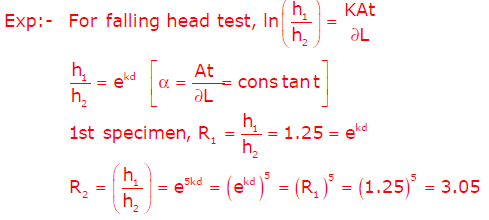QUESTION: 47

A layer of normally consolidated, saturated silty clay of 1 m thickness is subjected to one dimensional consolidation under a pressure increment of 20 kPa. The properties of the soil are: specific gravity = 2.7, natural moisture content = 45%, compression index = 0.45, and recompression index = 0.05. The initial average effective stress within the layer is 100 kPa. Assuming Terzaghi’s theory to be applicable, the primary consolidation settlement (rounded off to the nearest mm) is

Solution: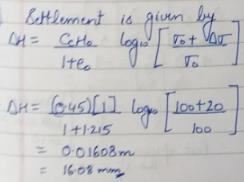QUESTION: 48

Steady state seepage is taking place through a soil element at Q, 2 m below the ground surface immediately downstream of the toe of an earthen dam as shown in the sketch. The water level in a piezometer installed at P, 500 mm above Q, is at the ground surface. The water level in a piezometer installed at R, 500 mm below Q, is 100 mm above the ground surface. The bulk saturated unit weight of the soil is 18 kN/m3 and the unit weight of water is 9.81 kN/m3. The vertical effective stress (in kPa) at Q is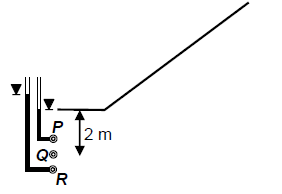Solution: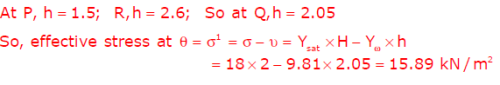QUESTION: 49

The top width and the depth of flow in a triangular channel were measured as 4 m and 1 m, respectively. The measured velocities on the centre line at the water surface, 0.2 m and 0.8 m below the surface are 0.7 m/s, 0.6 m/s and 0.4 m/s, respectively. Using two-point method of velocity measurement, the discharge (in m3/s) in the channel is

Solution: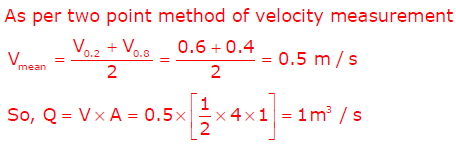QUESTION: 50

A water treatment plant is required to process 28800 m3/d of raw water (density = 1000 kg/m3, kinematic viscosity = 10-6 m2/s). The rapid mixing tank imparts tank a velocity gradient of 900s-1 to blend 35 mg/l of alum with the flow for a detention time of 2 minutes. The power input (W) required for rapid mixing is

Solution:
QUESTION: 51

Wheat crop requires 55 cm of water during 120 days of base period. The total rainfall during this period is 100 mm. Assume the irrigation efficiency to be 60%. The area (in ha) of the land which can be irrigated with a canal flow of 0.01 m3/s is

Solution:
QUESTION: 52

A water sample has a pH of 9.25. The concentration of hydroxyl ions in the water sample is

Solution: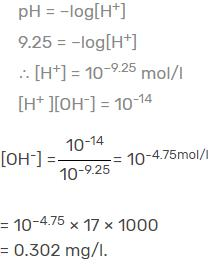QUESTION: 53

A town is required to treat 4.2 m3/min of raw water for daily domestic supply. Flocculating particles are to be produced by chemical coagulation. A column analysis indicated that an overflow rate of 0.2 mm/s will produce satisfactory particle removal in a settling basin at a depth of 3.5 m. The required surface area (in m2 ) for settling is

Solution: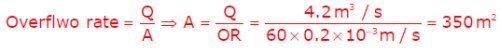QUESTION: 54

A pavement designer has arrived at a design traffic of 100 million standard axles for a newly developing national highway as per IRC:37 guidelines using the following data: design life = 15 years, commercial vehicle count before pavement construction = 4500 vehicles/day, annual traffic growth rate = 8%. The vehicle damage factor used in the calculation was

Solution: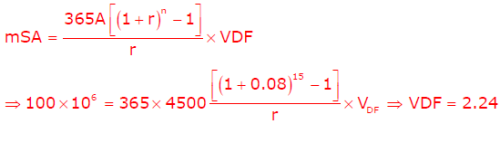QUESTION: 55

The following data are related to a horizontal curved portion of a two-lane highway: length of curve = 200 m, radius of curve = 300 m and width of pavement = 7.5 m. In order to provide a stopping sight distance (SSD) of 80 m, the set back distance (in m) required from the centre line of the inner lane of the pavement is

Solution:
QUESTION: 56

A two-lane urban road with one-way traffic has a maximum capacity of 1800 vehicles/hour. Under the jam condition, the average length occupied by the vehicles is 5.0 m. The speed versus density relationship is linear. For a traffic volume of 1000 vehicles/hour, the density (in vehicles/km) is

Solution:
QUESTION: 57

The horizontal distance between two stations P and Q is 100 m. The vertical angles from P and Q to the top of a vertical tower at T are 3° and 5° above horizontal, respectively. The vertical angles from P and Q to the base of the tower are 0.1° and 0.5° below horizontal, respectively. Stations P, Q and the tower are in the same vertical plane with P and Q being on the same side of T. Neglecting earth’s curvature and atmospheric refraction, the height (in m) of the tower is

Solution: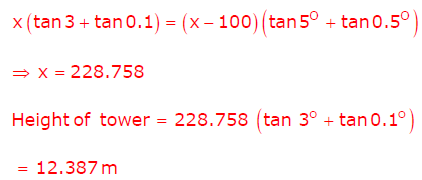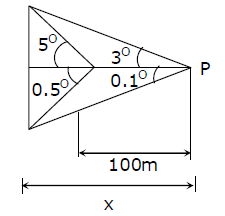QUESTION: 58

Common Data Questions
Common Data for Questions 58 and 59:

The flow net around a sheet pile wall is shown in the sketch. The properties of the soil are: permeability coefficient = 0.09 m/day (isotropic), specific gravity = 2.70 and void ratio = 0.85. The sheet pile wall and the bottom of the soil are impermeable.

The seepage loss (in m3 per day per unit length of the wall) of water is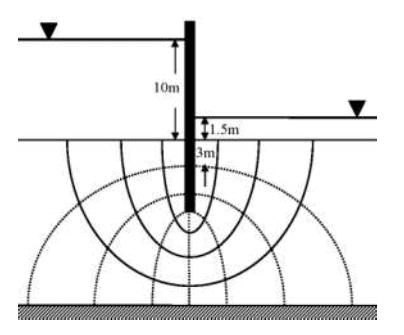Solution:
QUESTION: 59

The flow net around a sheet pile wall is shown in the sketch. The properties of the soil are: permeability coefficient = 0.09 m/day (isotropic), specific gravity = 2.70 and void ratio = 0.85. The sheet pile wall and the bottom of the soil are impermeable.

The factor of safety against the occurrence of piping failure isSolution:
QUESTION: 60

Common Data for Questions 60 and 61:
An activated sludge system (sketched below) is operating at equilibrium with the following information. Wastewater related data: flow rate = 500 m3/hour, influent BOD = 150 mg/L, effluent BOD = 10 mg/L. Aeration tank related data: hydraulic retention time = 8 hours, mean-cell-residence time = 240 hours, volume = 4000 m3, mixed liquor suspended solids = 2000 mg/L.

The food-to-biomass (F/M) ratio (in kg BOD per kg biomass per day) for the aeration tank is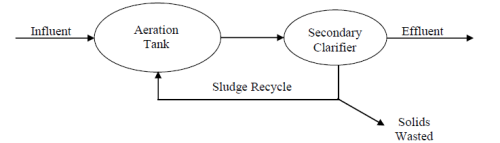Solution: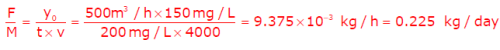QUESTION: 61

An activated sludge system (sketched below) is operating at equilibrium with the following information. Wastewater related data: flow rate = 500 m3/hour, influent BOD = 150 mg/L, effluent BOD = 10 mg/L. Aeration tank related data: hydraulic retention time = 8 hours, mean-cell-residence time = 240 hours, volume = 4000 m3, mixed liquor suspended solids = 2000 mg/L.

The mass (in kg/day) of solids wasted from the system isSolution: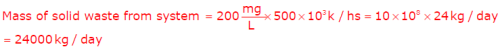QUESTION: 62

The cross-section at mid-span of a beam at the edge of a slab is shown in the sketch. A portion of the slab is considered as the effective flange width for the beam. The grades of concrete and reinforcing steel are M25 and Fe415, respectively. The total area of reinforcing bars (As) is 4000 mm2. At the ultimate limit state, xu denotes the depth of the neutral axis from the top fibre. Treat the section as under-reinforced and flanged (xu > 100 mm).

The value of xu (in mm) computed as per the Limit State Method of IS 456:2000 is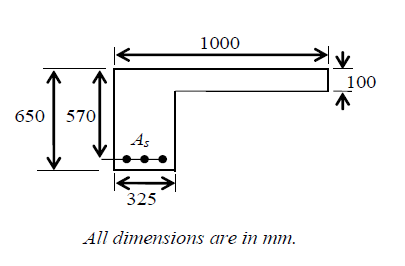Solution: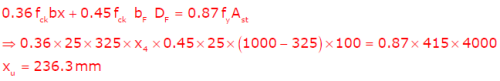QUESTION: 63

The cross-section at mid-span of a beam at the edge of a slab is shown in the sketch. A portion of the slab is considered as the effective flange width for the beam. The grades of concrete and reinforcing steel are M25 and Fe415, respectively. The total area of reinforcing bars (As) is 4000 mm2. At the ultimate limit state, xu denotes the depth of the neutral axis from the top fibre. Treat the section as under-reinforced and flanged (xu > 100 mm).

The ultimate moment capacity (in kNm) of the section, as per the Limit State Method of IS 456:2000 isSolution:
QUESTION: 64

The drainage area of a watershed is 50 km2. The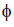index is 0.5 cm/hour and the base flow at the outlet is 10 m3/s. One hour unit hydrograph (unit depth = 1 cm) of the watershed is triangular in shape with a time base of 15 hours. The peak ordinate occurs at 5 hours.

The peak ordinate (in m3/s/cm) of the unit hydrograph is

Solution: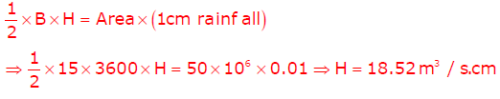QUESTION: 65

The drainage area of a watershed is 50 km2. Theindex is 0.5 cm/hour and the base flow at the outlet is 10 m3/s. One hour unit hydrograph (unit depth = 1 cm) of the watershed is triangular in shape with a time base of 15 hours. The peak ordinate occurs at 5 hours.

For a storm of depth of 5.5 cm and duration of 1 hour, the peak ordinate (in m3/s) of the hydrograph is

Solution: